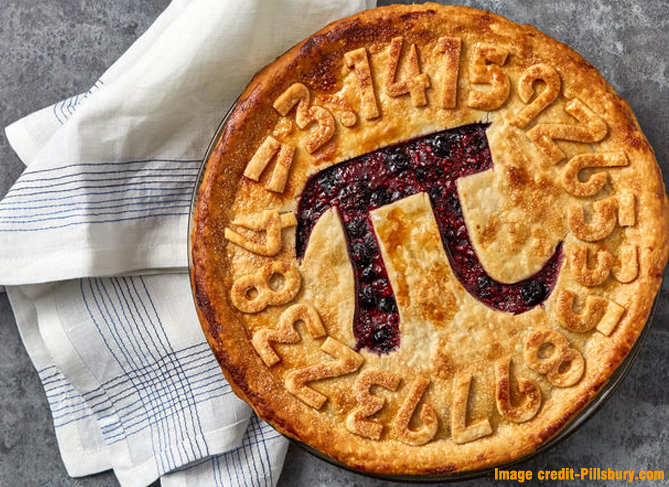# Pi Day on March 14: What this Day Means for Mathematicians and Fans of MathsThe first ten digits of Pi are 3.1415926535.The first ten digits of Pi are 3.1415926535.

The number π is related to mathematics; it explains the ratio of a circle’s circumference to its diameter. Pi appears in various formulas in all areas of mathematics and physics, and it is approximately equal to 3.14159. It represents the Greek letter π that has been used since the 18th century. Pi day is celebrated annually on March 14 (written 3/14 in the month/day format) because 3, 1, and 4 are the initial three-digit of π.

# When was Pi Day celebrated for the first time?

The first official Pi Day celebration took place on March 14, 1988, at the San Francisco Exploratorium. Noted physicist, Larry Shaw was the organiser of this event. This day got a boost when the US House of Representatives recognised March 14 as the Pi Day.

## Who holds the world record for memorising the digits of pi?

The first ten digits of Pi are 3.1415926535. How many digits can a man memorise? Suresh Kumar Sharma of India set the world record and memorised 70,030 digits in 2015.

## Did Archimedes calculate the value of pi?

We have to go back a few thousand years to know about this elusive number. Credit should be given to the noted mathematician of the ancient age Archimedes of Syracuse (287–212 BC) for calculating the value of pi. However, William Oughtred introduced pi with the Greek letters in 1647. It is regarded that Leonhard Euler used the pi symbol for the first time in 1737 into common use. But this view is debatable. Some say that William Jones, a close friend of Newton, for the first time used the symbol π, the Greek letter of the pi.

## What happens on Pi Day

On this day several programmes take place across the world with certain activities. Students try to solve typical mathematical problems. In educational institutions and science centres, pi memorisation challenge happens, numerical fun and quiz programmes are organised. Some of the dishes are named on pi such as pizza pie, fruit pie and so on. This celebration aims to enhance the interest of people in mathematics and physics.

### Some interesting facts of pi

• The pi symbol is being used for over 250 years.

• The exact value can’t be calculated.

• The people of Egypt believe that the pyramids of Giza were constructed on the principles of pi.

• The number pi is considered useful when it is used in calculations.

• Pi is a part of Egyptian mythology.

• Human beings are unable to find all the digits of pi due to its irrational number. Some of the ancient civilisations like Babylonian set the fraction 3 ⅛ while Chinese used the integer 3. However, Newton calculated pie to 16 decimal in 1665.